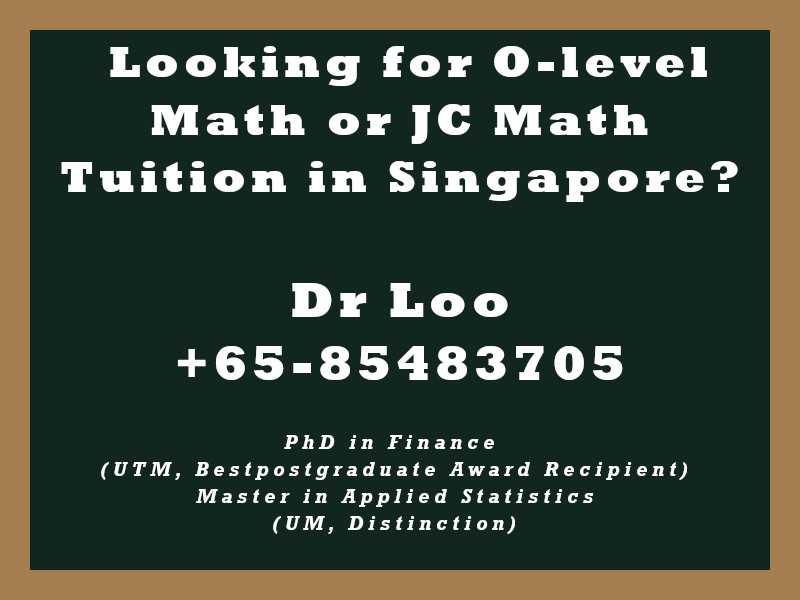+65-85483705
Select Page

# Standard Vector form equation of the plane

Question

1. What is the cartesion equation of a plane with vector equation equation to r.(3i + j – 2k) = 5
2. Determine the distance from the plane from origin for equation 3x + y – 2k = 51

1. 3x + y – 2k = 5
2. 51/√14

Note:

Cartesian equation of the plane

Ax+By+Cz = D

Standard vector form equation of the plane

r.n = D

You may follow this link if you are interested to find out the distance between a point and a plane.

## About the Math Tutor - Dr Loo

I am a PhD holder with 9 years of teaching experience at secondary school and university. My expertizes are mathematics, statistics, econometrics, finance and machine learning.

Currently I do provide consultation and private tuition in Singapore. For those who are looking for math tutor in Singapore or statistics tutor in Singapore, please feel free to contact me at +65-85483705 (SMS/Whatsapp/Telegram).

Some of the math tuition in Singapore I provide includes O-level math tuition and also JC Math Tuition (H1 Math Tuition & H2 Math Tuition). On the other hand, my statistics tuition in Singapore mostly focus on pre-university level until postgraduate level. For those who prefer online tutoring service, the online O-level math tuition and online JC math tuition are also available.## For those who are looking for math tution in Singapore

Need help with this topic? I do provide mathematics home tuition in Singapore for O-level math and also JC H2 math. In addition, online math tutoring is available as well. Feel free to contact me if you would like to know further.